Next: Model-based clustering Up: K-means Previous: K-means   Contents   Index

## Cluster cardinality in K-means

We stated in Section 16.2 that the number of clusters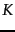is an input to most flat clustering algorithms. What do we do if we cannot come up with a plausible guess for?

A naive approach would be to select the optimal value ofaccording to the objective function, namely the value ofthat minimizes RSS. Defining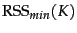as the minimal RSS of all clusterings withclusters, we observe thatis a monotonically decreasing function in(Exercise 16.7 ), which reaches its minimum 0 for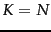where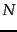is the number of documents. We would end up with each document being in its own cluster. Clearly, this is not an optimal clustering.

A heuristic method that gets around this problem is to estimateas follows. We first perform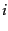(e.g.,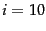) clusterings withclusters (each with a different initialization) and compute the RSS of each. Then we take the minimum of theRSS values. We denote this minimum by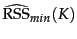. Now we can inspect the valuesasincreases and find the knee'' in the curve - the point where successive decreases in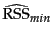become noticeably smaller. There are two such points in Figure 16.8 , one at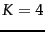, where the gradient flattens slightly, and a clearer flattening at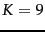. This is typical: there is seldom a single best number of clusters. We still need to employ an external constraint to choose from a number of possible values of(4 and 9 in this case).

A second type of criterion for cluster cardinality imposes a penalty for each new cluster - where conceptually we start with a single cluster containing all documents and then search for the optimal number of clustersby successively incrementingby one. To determine the cluster cardinality in this way, we create a generalized objective function that combines two elements: distortion , a measure of how much documents deviate from the prototype of their clusters (e.g., RSS for-means); and a measure of model complexity . We interpret a clustering here as a model of the data. Model complexity in clustering is usually the number of clusters or a function thereof. For-means, we then get this selection criterion for: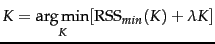(195)

where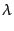is a weighting factor. A large value offavors solutions with few clusters. For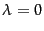, there is no penalty for more clusters andis the best solution.

The obvious difficulty with Equation 195 is that we need to determine. Unless this is easier than determiningdirectly, then we are back to square one. In some cases, we can choose values ofthat have worked well for similar data sets in the past. For example, if we periodically cluster news stories from a newswire, there is likely to be a fixed value ofthat gives us the rightin each successive clustering. In this application, we would not be able to determinebased on past experience sincechanges.

A theoretical justification for Equation 195 is the Akaike Information Criterion or AIC, an information-theoretic measure that trades off distortion against model complexity. The general form of AIC is: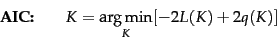(196)

where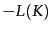, the negative maximum log-likelihood of the data forclusters, is a measure of distortion and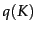, the number of parameters of a model withclusters, is a measure of model complexity. We will not attempt to derive the AIC here, but it is easy to understand intuitively. The first property of a good model of the data is that each data point is modeled well by the model. This is the goal of low distortion. But models should also be small (i.e., have low model complexity) since a model that merely describes the data (and therefore has zero distortion) is worthless. AIC provides a theoretical justification for one particular way of weighting these two factors, distortion and model complexity, when selecting a model.

For-means, the AIC can be stated as follows: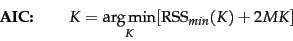(197)

Equation 197 is a special case of Equation 195 for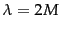.

To derive Equation 197 from Equation 196 observe that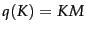in-means since each element of thecentroids is a parameter that can be varied independently; and that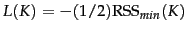(modulo a constant) if we view the model underlying-means as a Gaussian mixture with hard assignment, uniform cluster priors and identical spherical covariance matrices (see Exercise 16.7 ).

The derivation of AIC is based on a number of assumptions, e.g., that the data are . These assumptions are only approximately true for data sets in information retrieval. As a consequence, the AIC can rarely be applied without modification in text clustering. In Figure 16.8 , the dimensionality of the vector space is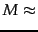50,000. Thus,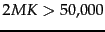dominates the smaller RSS-based term (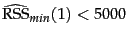, not shown in the figure) and the minimum of the expression is reached for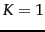. But as we know,(corresponding to the four classes China, Germany, Russia and Sports) is a better choice than. In practice, Equation 195 is often more useful than Equation 197 - with the caveat that we need to come up with an estimate for.

Exercises.

• Why are documents that do not use the same term for the concept car likely to end up in the same cluster in-means clustering?

• Two of the possible termination conditions for-means were (1) assignment does not change, (2) centroids do not change (page 16.4 ). Do these two conditions imply each other?Next: Model-based clustering Up: K-means Previous: K-means   Contents   Index